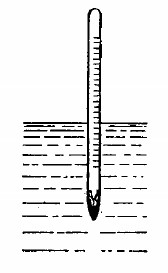# Calculate the period of small oscillations of a hydrometer (Fig) which was slightly pushed down in the vertical direction.The mass of the hydrometer i...

Calculate the period of small oscillations of a hydrometer (Fig) which was slightly pushed down in the vertical direction.The mass of the hydrometer is m = 50 g, the radius of its tube is r = 3.2 mm, the density of the liquid is p = 1.00 g/cm3. The resistance of the liquid is assumed to be negligible.Anonymous User Physics Oscillation 02 May, 2020 57 views